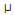# Electronics - Analog and Digital Converters

Exercise : Analog and Digital Converters - General Questions
1.
Which of the following is a type of error associated with digital-to-analog converters (DACs)?
nonmonotonic error
incorrect output codes
offset error
nonmonotonic and offset error
Explanation:
No answer description is available. Let's discuss.

2.
A 4-bit R/2R digital-to-analog (DAC) converter has a reference of 5 volts. What is the analog output for the input code 0101.
0.3125 V
3.125 V
0.78125 V
–3.125 V
Explanation:
No answer description is available. Let's discuss.

3.
A binary-weighted digital-to-analog converter has an input resistor of 100 k. If the resistor is connected to a 5 V source, the current through the resistor is:
50A
5 mA
500A
50 mA
Explanation:
No answer description is available. Let's discuss.

4.
What is the resolution of a digital-to-analog converter (DAC)?
It is the comparison between the actual output of the converter and its expected output.
It is the deviation between the ideal straight-line output and the actual output of the converter.
It is the smallest analog output change that can occur as a result of an increment in the digital input.
It is its ability to resolve between forward and reverse steps when sequenced over its entire range.